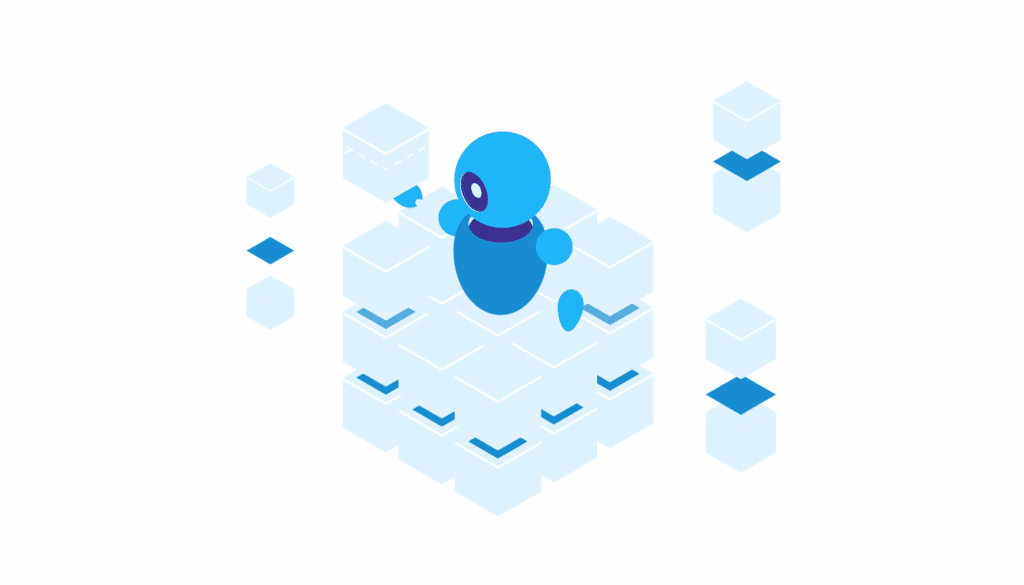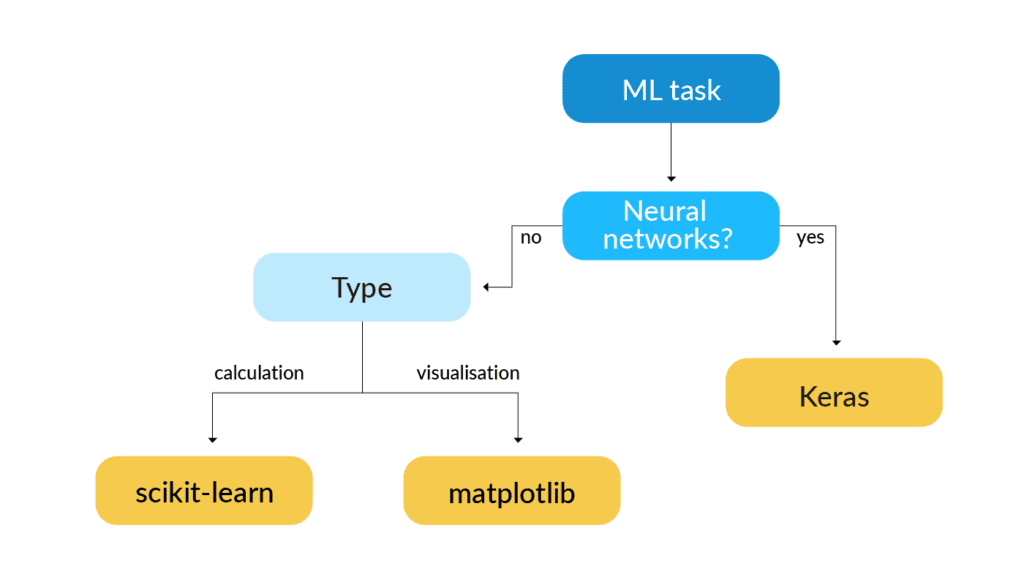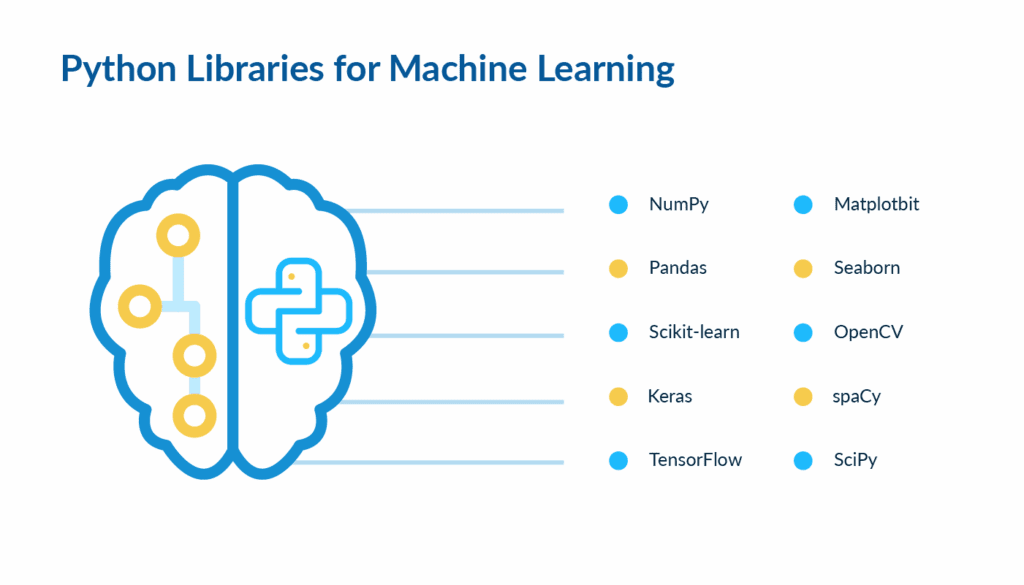# Python for Machine Learning

Python has been rapidly rising in popularity over the past few years. In 2019, the Stackoverflow survey reported Python to be the most wanted programming language and the second most loved one after Rust. The 2020 data from RedMonk indicates that Python is the second most popular language by the number of projects on GitHub. According to the TIOBE Index, Python has also ascended from its all-time low of 23rd place in 2000 to being the third most popular programming language in just twenty years.

Besides, Python is considered one of the best solutions when it comes to machine learning and data science. Below are a few examples of why Python is used for machine learning and how your business may benefit from using this flexible, productive, and convenient technology.

## Top Reasons Why Python is Used for Machine Learning Projects

#### Numerous Libraries

By itself, Python is an ordinary interpreted programming language. It’s not lightning fast compared to languages that are compiled, and it’s incredibly high-level, which makes it unsuitable for many objectives.The true strength of Python lies in an enormous number of machine learning libraries available for different tasks. The toolkit that Python offers to machine learning developers is extremely customizable and extensive. Not only do Python libraries allow developers to work more efficiently, but they also enable companies to hire employees with the skillset that corresponds precisely to the company’s needs. Likewise, developers can become proficient in many libraries but devote the greatest amount of attention to the ones that are most useful in their line of work.

#### Less Time Spent Coding from Scratch

The number of machine learning tools that Python provides in various libraries allows developers to cut the time they spend coding from scratch. While the ability to program a required functionality by hand is important and can indicate a developer’s proficiency, it’s incredibly time-consuming to do it in production.Python grants access to already written machine learning algorithms and frameworks that can be applied to any project. Developers, therefore, spend more time designing the architecture, establishing the logic, and optimizing the application. Compared to other languages, Python gives developers the greatest ability to use the tools rather than build them first.

#### Wide Range of Applications and Prototype Building

Python can be used for the most varied tasks, which makes it essential for many companies that don’t specialize in machine learning. The ease of use and the variety of external frameworks and libraries that interact with Python allow developers to use the language for data science, building cloud services, and even desktop and mobile application development.Also, developers frequently use Python when it’s necessary to build a prototype of an application first. Since the language is highly readable and easily learned, it consumes less time and other resources to design a draft of an app for presentation. Python allows assessing the functionality, effectiveness, and looks of a program before implementing it in a different language.

#### Huge Community

The fourth pillar of Python is its community. Since the distribution of various tools for this language is massive, and most of the libraries are open-source, the community support behind Python is incredibly strong. Businesses can benefit from it because it helps developers achieve machine learning mastery with Python faster and implement their machine learning solutions without a hitch. Also, having a big dedicated community means any issues that arise can be resolved quickly.## Machine Learning Algorithms in Python

#### Linear Regression

Linear regression is a supervised learning algorithm. It is used to predict a continuous rather than a categorical value, based on the already available labeled data. Simple linear regression produces a prediction depending on a single selected feature, while multiple regression accounts for more than one independent variable. Practically, the algorithm can be used to predict the price of a house in a certain area, CO2 car emissions of a new vehicle, and so on.

Linear regression aims to fit a line through all data points in a way that would minimize the distance between each given point and the line. Although this algorithm is relatively easy to implement by coding from scratch, it’s time-consuming and often unnecessary. In Python, linear regression algorithms are contained in various ML packages. For instance, in the scikit-learn linear regression module, the logic is already written and can be applied to any dataset.

#### Decision Trees

The decision tree algorithm is a supervised machine learning model that can be used both for regression and classification tasks. This model has a flowchart-like structure, in which every decision node represents a test of subsequent attributes’ properties. The branches of a decision tree represent the outcomes of such tests. End nodes, also called leaf nodes, represent final decisions that are generated after processing all attributes.In Python, the decision tree algorithm can be implemented in three main steps. First of all, the root node needs to be selected from all the attributes. Then, the remaining training dataset has to be split into subsets. The process is repeated recursively on all subsets until there are no more attributes left.

Decision trees in Python can be implemented using models from the scikit-learn ML library.

#### K-means Clustering

K-means is an unsupervised clustering algorithm. It works on unlabeled data and aims to divide it into a given number of clusters by certain similar characteristics. The model first trains on a dataset, creating a number of clusters. It should then produce one of the clusters’ names as the output to any given test data point.

To implement the k-means algorithm in Python, you need to decide on the number of clusters, k, that the data should be grouped in. The algorithm should then find k centroids in the data, or one centroid per cluster. Then, the algorithm must start assigning each point in the remaining data to a certain cluster based on what centroid it’s closest to in value. All the centroids are subsequently recalculated, and the process is repeated until no centroid changes its value upon the adjustment.

Scikit-learn provides the k-means clustering algorithm for Python. Other libraries, such as Matplotlib or Seaborn, can be used to visualize the outcome of the computation.

## Python Libraries for Machine Learning

Below is a brief description of several libraries and tools most commonly used to build machine learning applications with Python. All code samples can be found here.#### NumPy

NumPy is a Python library for scientific computations. The package is primarily used to perform mathematical operations and structure any given data in a way that allows developers to work with it more efficiently. NumPy presents random number generation capabilities, as well as linear algebra tools, the Fourier transform, and extensive multidimensional array manipulation toolkit.

Also, NumPy operates faster than native Python lists.

```import time start.time = time.time ( ) list_size = [0,0,0,0,0,0,0,0,0,0,0,0,0,0,0,0,0,0,0,0] end.time = time.time ( ) print. (end_time - start_time) ```

```0,00022363662719726562 ```

In the code sample above, the output is the time elapsed between the beginning and the end of the creation of a native Python list. It demonstrates a fairly small array, but NumPy performs faster even on this scale:

```start_time = time.time() a = np.zeros((20)) end_time = time.time() print(end_time - start_time)```

np.zeros creates an array of all zeros with specified dimensions. NumPy is also integrated into other libraries for machine learning, which makes it particularly functional for this purpose.

#### Pandas

While NumPy can be used to arrange and manipulate data, pandas is one of the machine learning Python packages used to analyze it. It is particularly useful in the development of a machine learning application, as it relies heavily upon the quality of the initial input. Pandas is known for its fast and efficient DataFrame object, the capabilities to read and write data in different formats, including CSV files, SQL databases, Microsoft Excel, and in-memory data structures.

#### Scikit-learn

Scikit-learn is a library that contains the main Python machine learning algorithms that programmers use. It allows developers to utilize regression, classification, and clustering algorithms to perform the required operation or prediction just in a few lines of code. The library features such ML Python algorithms as k-means, random forests, gradient boosting, support vector machines, linear regression, and several others.

Scikit-learn also provides datasets for learning, which can be useful in employee training:

```import numpy as np from sklearn import datasets, linear_model from sklearn.model_selection import train_test_split x_value, y_value = datasets.load_diabetes(return_X_y=True) ```

In this sample machine learning code, we want to create a simple linear regression model, and hence we only need to use one independent feature:

```x_value = x_value[:, np.newaxis, 2] ```

Then, scikit-learn allows us to split the data into a training and a test sets randomly:

```x_train, x_test, y_train, y_test = train_test_split(x_value, y_value, test_size=0.31) ```

After this, we can create and train a model without coding the logic behind the calculations explicitly:

```regression_example = linear_model.LinearRegression() regression_example.fit(x_train, y_train) ```

Finally, we can generate a prediction:

```gen_prediction = regression_example.predict(x_test) ```

#### Keras

Keras is an API that can run on top of Thano, TensorFlow, and the Microsoft Cognitive Toolkit. It was created to enable developers to experiment with neural networks and implement the programmers’ ideas faster. Keras prides itself on being human-friendly and on improving developers’ performance by simplifying the process of writing the code. The API supports convolutional networks and recurrent networks, runs on both CPU and GPU, and, most importantly, allows fast prototyping.

#### TensorFlow

TensorFlow is not just a library but a whole platform consisting of Python packages for machine learning. TensorFlow collects all resources necessary to build an ML application quickly and efficiently. The toolkit is frequently compared to Keras and scikit-learn, although its target functionality is slightly different from the two. TensorFlow works best when applied to deep neural networks research and machine learning.

#### Matplotlib

Matplotlib is one of the most widely known machine learning Python libraries for data visualization. It can be used to create static, dynamic, and interactive graphs, charts, and other forms of visual data representation. Matplotlib is generally highly customizable.

In the scientific field, data science and machine learning with Python are hardly possible without data visualization. Businesses can utilize it for presentations and making the outputs of machine learning algorithms more readable.

```import matplotlib plt.plot([1, 2, 3], [2, -1, 5]) plt.show() ```

Matplotlib enables you to create a simple but descriptive graph in as little as two lines of code. A bit more complex one may add specific labels, transparency, and type of the chart as needed:

```import matplotlib.pyplot as plt sizes = [13, 23, 40, 10, 14] labels = 'Post-doc', 'PhD', 'High school', 'Undergraduate', 'Masters' a, ax1 = plt.subplots() ax1.pie(sizes, explode=(0, 0.1, 0, 0.1, 0), labels=labels, autopct='%1.f%%', shadow=True, startangle=90) ax1.axis('equal') plt.show() ```

Here, sizes defines the percentages displayed on each slice of the pie chart counterclockwise. Labels mark the respective pieces. The autopct parameter defines how floating-point numbers will be handled; startangle and ax1.axis define the disposition of the graph.

#### Seaborn

Seaborn is another data visualization library. It’s based on Matplotlib, and it presents an upgraded version of the latter. Seaborn has more default themes and can be applied for a wider range of objectives than Matplotlib is.

#### OpenCV

OpenCV is a library containing approximately 2500 optimized algorithms for Python AI programming, computer vision and other types of machine learning. It is distributed under BSD license, which means that businesses have almost no restrictions in using it. Companies can utilize OpenCV to detect and identify faces and objects, track camera movements, classify actions in videos, improve the resolution of an image, and find similar images from any database. This Python machine learning package is incredibly useful in the creation of augmented reality applications.

#### spaCy

spaCy is an AI and machine learning Python library for natural language processing. It was designed specifically to be implemented in commercial products, and it’s the software under the MIT license. SpaCy features pre-trained statistical models, as well as the support for more than fifty languages, convolutional neural networks, admirable speed, and deep learning integration.

```import spacy nlp = space.load("en_core_web_sm") doc = nlp("Robots will win") robots = doc will = doc win = doc ```

This small machine learning code example demonstrates the most basic of spaCy’s capabilities. It defines which part of speech any word in the given sentence is. The result is as follows:

```print("The word 'robots' is a ", robots.pos_) print("The word 'will' is a ", will.pos_) print("The word 'win' is a ", win.pos_)```

#### SciPy

SciPy is an ecosystem, based on various Python tools for scientific computations and machine learning. One of its essential elements is the SciPy library. The library contains modules and algorithms for linear algebra, statistics, optimization, image processing, and interpolation. The SciPy stack contains the NumPy package, as well as Matplotlib, pandas, and SymPy, in addition to many others.

## Wrapping Up

Python is one of the most popular programming languages for machine learning. It’s easy to use, highly readable, and incredibly functional due to the wide variety of libraries that it supports. Companies can benefit from using this language, as Python can be used both in application development and machine learning.

The language can also boost developers’ productivity since it removes the necessity to code basic algorithms from scratch. Machine learning relies upon a variety of well-known algorithms to function, such as linear regression, random trees, k-means, and many others. Machine learning libraries in Python, such as scikit-learn and TensorFlow, contain these algorithms as ready-to-use functions.

The reduction of time required to write the code, the ease of use, and the extensive choice of instruments tailored for specific tasks all make Python the language of choice for data scientists and companies that employ machine learning to help their businesses grow. LITSLINK, as a leading Python development company and trusted software development services provider, is always here to turn your ML ideas into profitable ventures.